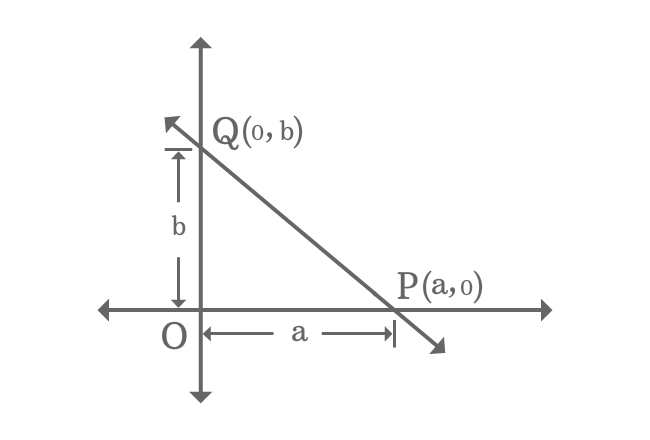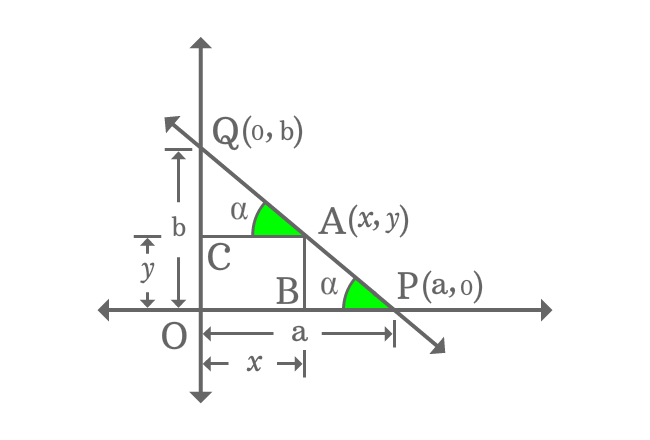# Intercept form of a Line

## Equation

$\dfrac{x}{a}+\dfrac{y}{b} \,=\, 1$

It is an equation of a straight line when a straight line intersects both axes with $x$ and $y$ intercepts.

### ProofA straight line intersects both horizontal and vertical axes of Cartesian coordinate system at $P$ and $Q$ points with $a$ as $x$-intercept and $b$ as $y$-intercept.

The intersecting points of straight line $\small \overleftrightarrow{PQ}$ in coordinate form are $P(a, 0)$ and $Q(0, b)$.

$A(x, y)$ is any point on the straight line $\small \overleftrightarrow{PQ}$. Draw a perpendicular line to $x$-axis from point $A$ and it intersects the $x$-axis at point $B$.

Similarly, draw a perpendicular line to $y$-axis from point $A$ and it intersects the $y$-axis at point $C$.

It formed two right triangles $\Delta APB$ and $\Delta QAC$. The adjacent side of $\Delta APB$ is on $x$-axis and the adjacent side of $\Delta QAC$ is parallel to the $x$-axis. Similarly, the opposite side of $\Delta QAC$ is on $y$-axis and the opposite side of $\Delta APB$ is parallel to the $y$-axis. The hypotenuse of both right triangles are part of straight line $\small \overleftrightarrow{PQ}$.Therefore, the right triangles $\Delta APB$ and $\Delta QAC$ are similar triangles. Hence, the corresponding angles of both triangles are same.

If $\angle QAC$ is equal to $\alpha$, then $\angle APB$ is also equal to $\alpha$.

According to $\Delta APB$

$\tan{\alpha} \,=\, \dfrac{AB}{BP}$

$\implies \tan{\alpha} \,=\, \dfrac{AB}{OP-OB}$

$\,\,\, \therefore \,\,\,\,\,\, \tan{\alpha} \,=\, \dfrac{y}{a-x}$

According to $\Delta QAC$

$\tan{\alpha} \,=\, \dfrac{QC}{AC}$

$\implies \tan{\alpha} \,=\, \dfrac{OQ-OC}{AC}$

$\,\,\, \therefore \,\,\,\,\,\, \tan{\alpha} \,=\, \dfrac{b-y}{x}$

According to both $\Delta APB$ and $\Delta QAC$

$\tan{\alpha} \,=\, \dfrac{y}{a-x} \,=\, \dfrac{b-y}{x}$

Now, simplify the equation to get the equation of a straight line in intercept form.

$\dfrac{y}{a-x} \,=\, \dfrac{b-y}{x}$

$\implies xy \,=\, (a-x)(b-y)$

$\implies xy \,=\, a(b-y)-x(b-y)$

$\implies xy \,=\, ab-ay-xb+xy$

$\implies \require{cancel} \cancel{xy} \,=\, ab-ay-xb+\cancel{xy}$

$\implies 0 \,=\, ab-ay-xb$

$\implies bx+ay \,=\, ab$

$\implies \dfrac{bx+ay}{ab} \,=\, 1$

$\implies \dfrac{bx}{ab}+\dfrac{ay}{ab} \,=\, 1$

$\implies \require{cancel} \dfrac{\cancel{b}x}{a\cancel{b}}+\dfrac{\cancel{a}y}{\cancel{a}b} \,=\, 1$

$\,\,\, \therefore \,\,\,\,\,\, \dfrac{x}{a}+\dfrac{y}{b} \,=\, 1$

It is a linear equation in algebraic form and represents an equation of straight line when a straight line intersects both axes with double intercept.

Latest Math Topics
Jun 26, 2023
Jun 23, 2023

Latest Math Problems
Jul 01, 2023
Jun 25, 2023
###### Math Questions

The math problems with solutions to learn how to solve a problem.

Learn solutions

Practice now

###### Math Videos

The math videos tutorials with visual graphics to learn every concept.

Watch now

###### Subscribe us

Get the latest math updates from the Math Doubts by subscribing us.